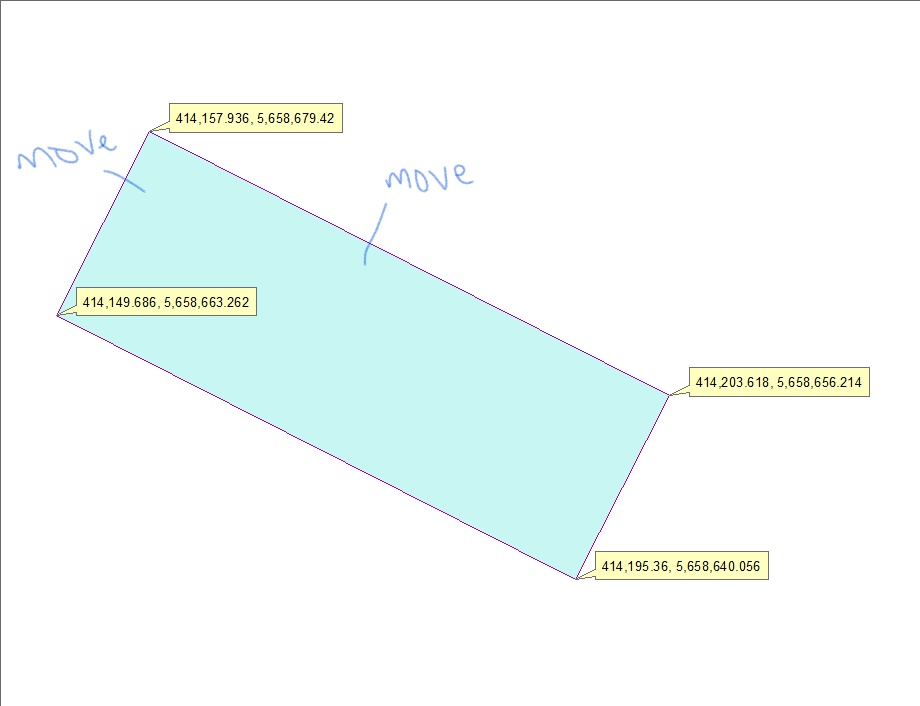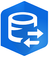# rotate split polylines python arcpy

1623
10
02-24-2020 12:36 PMNew Contributor II

I have converted polygon edges into polylines which I have also split at vertices. I am trying to rotate the polygon edges (segments) at angles which I have stored in a field in an attribute table.

The angles I have are for the polygon vertices. How do I proceed with rotating the segments -- preferably by scripting?For instance, in the above image with a polygon, I need to move those 2 line segments until the angle between them are at right-angle and repeat same for all vertex/corners of the polygon.

Tags (4)
1 Solution

Accepted SolutionsbyMVP Legendary Contributor

Moving isn't the method.  You have to pick the long side that will remain stationary, determine the short edge lengths and decide which you will use and derive its length.  From the end points of the fixed long side and the desired edge length, you need to calculate the perpendicular offset points and replace the 'bad' long side points with these new values.  You will then have a rectangle.  You could do this iteratively, but that would be a wasted effort.

If these were constructed, make sure that the construct polygons with right angles is used.... 90 degrees all the time

10 RepliesbyMVP Regular Contributor

Dan Patterson Has an interesting solution here. You can also check out this solution for rotating polygons as it should be the same. Here's another discussion That might be helpful. In short, there doesn't appear to be a single tool that will rotate a vector feature. Another discussion proposed converting the feature to a raster and using the Rotate tool, then converting back to a vector shape but I you'd lose precision along the way.New Contributor II

Thank you, Blake. I am going through each now.byEsri Regular Contributor

I can't quite picture what you're looking to do but making squared off boundaries and rotating features is in this sample:New Contributor II

Thank you, Bruce, for the link. But not sure that gets me to my goal.

Let me attempt to say it differently. So, the screenshot in the OP is a building footprint and the angles at the vertex aren't 90 degrees but should be -- digitizing error. I am now trying to adjust each corner which has angles such as 89.88 degrees to become 90 degrees. I am honestly not sure how to go about it and so I am in search of a solution.byEsri Regular Contributor

OK!

Requires 3D Analyst extension.New Contributor II

Thank you for this, Bruce. I am indeed aware of this tool but the current requirement is to script such a solution.byMVP Legendary Contributor

Moving isn't the method.  You have to pick the long side that will remain stationary, determine the short edge lengths and decide which you will use and derive its length.  From the end points of the fixed long side and the desired edge length, you need to calculate the perpendicular offset points and replace the 'bad' long side points with these new values.  You will then have a rectangle.  You could do this iteratively, but that would be a wasted effort.

If these were constructed, make sure that the construct polygons with right angles is used.... 90 degrees all the timeNew Contributor II

Absolutely spot on, Dan. This was my first thought line and I could get the lengths of the polygon sides using:

``````arcpy.MinimumBoundingGeometry_management(inFC, outFC,
geometry_type="RECTANGLE_BY_AREA",
group_option="NONE",
mbg_fields_option=True)‍‍‍‍‍‍‍‍‍‍‍‍``````

The length and even orientation were computed after executing the above with split polylines (edges of the polygon) as the input Feature class. From there I wasn't sure what else I should be doing. Do you select the side with the longest line and then draw the perpendicular offsets at a static value or from the width values? I'm really not sure how to go about this.

PS: The screenshot in the OP is one sample.byMVP Legendary Contributor

Your friend einsum....  The lengths are in sequential clockwise order from the start point (you can always determine/produce polygon points either as a separate feature class or values in a table.)

``````def lengths(poly):
"""Polyline lengths or polygon perimeter."""
def _cal(a):
"""Perform the calculation, mini-e_leng."""
diff = a[:-1] - a[1:]
return np.sqrt(np.einsum('ij,ij->i', diff, diff))
return _cal(poly)

[lengths(p) for p in [b1, b2, b3, b4]]

[array([ 10.0,  10.0,  10.0,  10.0]),
array([ 10.0,  11.2,  11.2]),
array([ 11.2,  10.0,  11.2]),
array([ 11.2,  11.2,  10.0])]

# ---- If you want to experiment
b1
array([[ 10.0,  0.0],
[ 10.0,  10.0],
[ 20.0,  10.0],
[ 20.0,  0.0],
[ 10.0,  0.0]])

b2
array([[ 20.0,  0.0],
[ 20.0,  10.0],
[ 30.0,  5.0],
[ 20.0,  0.0]])

b3
array([[ 15.0,  20.0],
[ 20.0,  10.0],
[ 10.0,  10.0],
[ 15.0,  20.0]])

b4
array([[ 10.0,  0.0],
[ 0.0,  5.0],
[ 10.0,  10.0],
[ 10.0,  0.0]])
‍‍‍‍‍‍‍‍‍‍‍‍‍‍‍‍‍‍‍‍‍‍‍‍‍‍‍‍‍‍‍‍‍‍‍‍‍‍‍‍‍``````